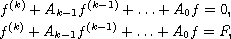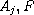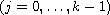Electron. J. Diff. Equ., Vol. 2014 (2014), No. 125, pp. 1-11.

### Growth of meromorphic solutions of higher order linear differential equations Lijun Wang, Huifang Liu

Abstract:
In this article, we investigate the growth of meromorphic solutions of the differential equationswhereare meromorphic functions. When there exists one dominant coefficient with lower order less than 1/2, we obtain some estimations of the hyper order and the hyper convergence exponent of zeros of meromorphic solutions of the above equations.

Submitted January 27, 2014. Published May 14, 2014.
Math Subject Classifications: 30D35, 39B12.
Key Words: Meromorphic function; differential equations; growth; order.

Show me the PDF file (241 KB), TEX file, and other files for this article.Lijun Wang College of Mathematics and Information Science Jiangxi Normal University, Nanchang 330022, China email: lijunwangz@163.com Huifang Liu College of Mathematics and Information Science Jiangxi Normal University, Nanchang 330022, China email: liuhuifang73@sina.com, 925268196@qq.com